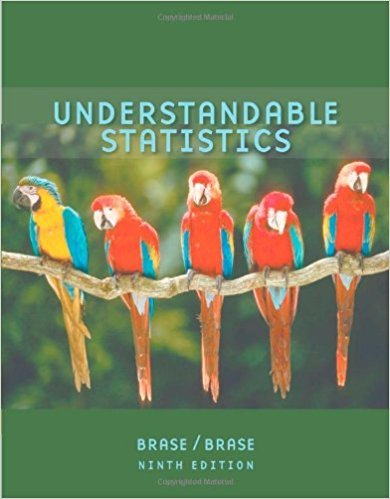×
×

# Solutions for Chapter 8: ESTIMATION## Full solutions for Understandable Statistics | 9th Edition

ISBN: 9780618949922Solutions for Chapter 8: ESTIMATION

Solutions for Chapter 8
4 5 0 424 Reviews
19
2
##### ISBN: 9780618949922

Understandable Statistics was written by and is associated to the ISBN: 9780618949922. Chapter 8: ESTIMATION includes 19 full step-by-step solutions. This textbook survival guide was created for the textbook: Understandable Statistics, edition: 9. Since 19 problems in chapter 8: ESTIMATION have been answered, more than 35325 students have viewed full step-by-step solutions from this chapter. This expansive textbook survival guide covers the following chapters and their solutions.

Key Statistics Terms and definitions covered in this textbook

A formula used to determine the probability of the union of two (or more) events from the probabilities of the events and their intersection(s).

• Attribute

A qualitative characteristic of an item or unit, usually arising in quality control. For example, classifying production units as defective or nondefective results in attributes data.

• Backward elimination

A method of variable selection in regression that begins with all of the candidate regressor variables in the model and eliminates the insigniicant regressors one at a time until only signiicant regressors remain

• Central composite design (CCD)

A second-order response surface design in k variables consisting of a two-level factorial, 2k axial runs, and one or more center points. The two-level factorial portion of a CCD can be a fractional factorial design when k is large. The CCD is the most widely used design for itting a second-order model.

• Central limit theorem

The simplest form of the central limit theorem states that the sum of n independently distributed random variables will tend to be normally distributed as n becomes large. It is a necessary and suficient condition that none of the variances of the individual random variables are large in comparison to their sum. There are more general forms of the central theorem that allow ininite variances and correlated random variables, and there is a multivariate version of the theorem.

• Conidence interval

If it is possible to write a probability statement of the form PL U ( ) ? ? ? ? = ?1 where L and U are functions of only the sample data and ? is a parameter, then the interval between L and U is called a conidence interval (or a 100 1( )% ? ? conidence interval). The interpretation is that a statement that the parameter ? lies in this interval will be true 100 1( )% ? ? of the times that such a statement is made

• Contingency table.

A tabular arrangement expressing the assignment of members of a data set according to two or more categories or classiication criteria

• Control limits

See Control chart.

• Cook’s distance

In regression, Cook’s distance is a measure of the inluence of each individual observation on the estimates of the regression model parameters. It expresses the distance that the vector of model parameter estimates with the ith observation removed lies from the vector of model parameter estimates based on all observations. Large values of Cook’s distance indicate that the observation is inluential.

• Correlation

In the most general usage, a measure of the interdependence among data. The concept may include more than two variables. The term is most commonly used in a narrow sense to express the relationship between quantitative variables or ranks.

• Cumulative distribution function

For a random variable X, the function of X deined as PX x ( ) ? that is used to specify the probability distribution.

• Density function

Another name for a probability density function

• Design matrix

A matrix that provides the tests that are to be conducted in an experiment.

• Distribution free method(s)

Any method of inference (hypothesis testing or conidence interval construction) that does not depend on the form of the underlying distribution of the observations. Sometimes called nonparametric method(s).

• Error of estimation

The difference between an estimated value and the true value.

• F-test

Any test of signiicance involving the F distribution. The most common F-tests are (1) testing hypotheses about the variances or standard deviations of two independent normal distributions, (2) testing hypotheses about treatment means or variance components in the analysis of variance, and (3) testing signiicance of regression or tests on subsets of parameters in a regression model.

• First-order model

A model that contains only irstorder terms. For example, the irst-order response surface model in two variables is y xx = + ?? ? ? 0 11 2 2 + + . A irst-order model is also called a main effects model

• Fisher’s least signiicant difference (LSD) method

A series of pair-wise hypothesis tests of treatment means in an experiment to determine which means differ.

• Fixed factor (or fixed effect).

In analysis of variance, a factor or effect is considered ixed if all the levels of interest for that factor are included in the experiment. Conclusions are then valid about this set of levels only, although when the factor is quantitative, it is customary to it a model to the data for interpolating between these levels.

• Frequency distribution

An arrangement of the frequencies of observations in a sample or population according to the values that the observations take on

×